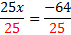# Clearing Equations of Decimals

 When equations have lots of decimals, like the one seen below, you may be able to solve it as it is written, but it will probably be easier to clear the decimals first. 0.25x + 0.35 = -0.29 To clear an equation of decimals, multiply each term on both sides by the power of ten that will make all the decimals whole numbers. In our example above, if we multiply .25 by 100, we will get 25, a whole number. Since each decimal only goes to the hundredths place, 100 will work for all three terms. So let's multiply each term by 100 to clear the decimals: (100)0.25x + (100)0.35 = (100)(-0.29) 25x + 35 = -29 Now we can solve the equation as normal: 25x + 35 - 35 = -29 - 35 25x = -64x = -2.56 Since the original was in decimal form, the answer should most likely also be in decimal form. Let's look at one more: 1.75x + 4 = 6.2 We have to think a little more carefully about what multiple of ten to use here. 6.2 only needs to be multiplied by 10, but 1.25 needs 100, so we will multiply every term by 100. Don't forget to multiply the 4 by 100 as well. (100)(1.75x) + (100)(4) = (100)(6.2) 175x + 400 = 620 We had to be extra careful as we multiplied by 100. Now we can solve the equation as normal: 175x + 400 - 400 = 620 - 400 175x = 220x = 1.26 Practice:Clear each equation of decimals, then solve. Round each answer to the hundredths place. 1) 0.2x + 3.5 = 8.8 2) 2.67y - 1.4 = 3.88 3) 4.2x + 3.3x - 1.1 = 4.7 4) 3.45x + 2.7 = 5 5) -2.5a + 4.67 = 2.881 Answers: 1) 26.5 2) 1.98 3) 0.77 4) 0.67 5) 0.72

 Related Links: Math Algebra Factors